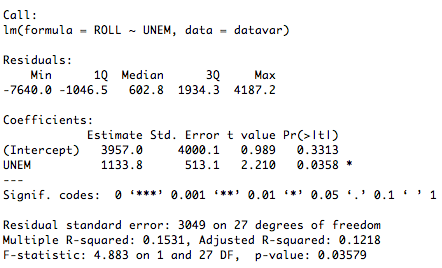Want to share your content on R-bloggers? click here if you have a blog, or here if you don't.

Simple linear regression uses a solitary independent variable to predict the outcome of a dependent variable. By understanding this, the most basic form of regression, numerous complex modeling techniques can be learned. This tutorial will explore how R can be used to perform simple linear regression.

### Tutorial Files

Before we begin, you may want to download the sample data (.csv) used in this tutorial. Be sure to right-click and save the file to your R working directory. This dataset contains information used to estimate undergraduate enrollment at the University of New Mexico (Office of Institutional Research, 1990). Note that all code samples in this tutorial assume that this data has already been read into an R variable and has been attached.

### Creating A Linear Model

#### The lm() function

In R, the lm(), or “linear model,” function can be used to create a simple regression model. The lm() function accepts a number of arguments (“Fitting Linear Models,” n.d.). The following list explains the two most commonly used parameters.

• formula: describes the model
• Note that the formula argument follows a specific format. For simple linear regression, this is “YVAR ~ XVAR” where YVAR is the dependent, or predicted, variable and XVAR is the independent, or predictor, variable.

• data: the variable that contains the dataset

It is recommended that you save a newly created linear model into a variable. By doing so, the model can be used in subsequent calculations and analyses without having to retype the entire lm() function each time. The sample code below demonstrates how to create a linear model and save it into a variable. In this particular case, we are using the unemployment rate (UNEM) to predict the fall enrollment (ROLL).

1. > #create a linear model using lm(FORMULA, DATAVAR)
2. > #predict the fall enrollment (ROLL) using the unemployment rate (UNEM)
3. > linearModelVar <- lm(ROLL ~ UNEM, datavar)
4. > #display linear model
5. > linearModelVar

The output of the preceding function is pictured below.From this output, we have determined that the intercept is 3957 and the coefficient for the unemployment rate is 1134. Therefore, the complete regression equation is Fall Enrollment = 3957 + 1134 * Unemployment Rate. This equation tells us that the predicted fall enrollment for the University of New Mexico will increase by 1134 students for every one percent increase in the unemployment rate. Suppose that our research question asks what the expected fall enrollment is, given this year’s unemployment rate of 9%. As follows, we can use the regression equation to calculate the answer to this question.

1. > #what is the expected fall enrollment (ROLL) given this year’s unemployment rate (UNEM) of 9%
2. > 3957 + 1134 * 9
3.  14163
4. > #the predicted fall enrollment, given a 9% unemployment rate, is 14,163 students.

#### Summarizing The Model

Naturally, simple linear regression can be used to do much more than just calculate expected values. Here, the summary(OBJECT) function is a useful tool. It is capable of generating most of the statistical information that one would need to derive from a linear model. The example below demonstrates the use of the summary function on a linear model variable.

1. > #use summary(OBJECT) to display information about the linear model
2. > summary(linearModelVar)

The output of the preceding function is pictured below.The summary(OBJECT) function has provided us with a wealth of information, including t-test, F-test, R-squared, residual, and significance values. All of this data can be used to answer important research questions related to our linear model. Yet again, the summary(OBJECT) function proves to be a valuable resource. It is worth remembering and using when conducting a variety of analyses in R.

#### Alternative Modeling Options

Although lm() was used in this tutorial, note that there are alternative modeling functions available in R, such as glm() and rlm(). Depending on your unique circumstances, it may be beneficial or necessary to investigate alternatives to lm() before choosing how to conduct your regression analysis.

### Complete Simple Linear Regression Example

To see a complete example of how simple linear regression can be conducted in R, please download the simple linear regression example (.txt) file.

### References

Fitting Linear Models. (n.d.). Retrieved November 22, 2009 from http://sekhon.berkeley.edu/library/stats/html/lm.html

Office of Institutional Research (1990). Enrollment Forecast [Data File]. Retrieved November 22, 2009 from http://lib.stat.cmu.edu/DASL/Datafiles/enrolldat.html